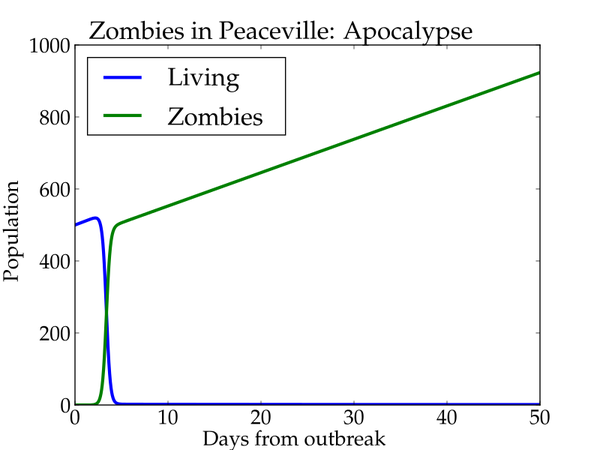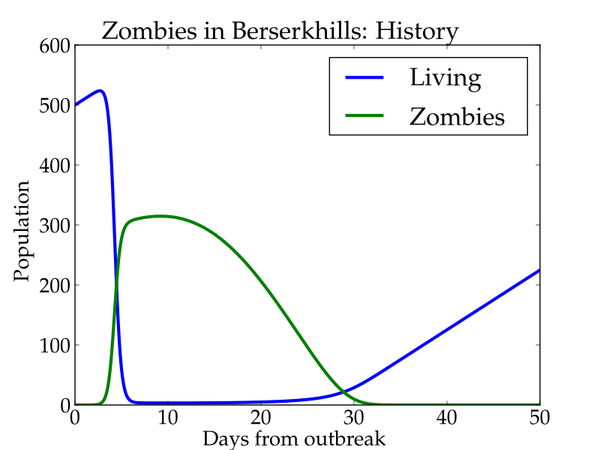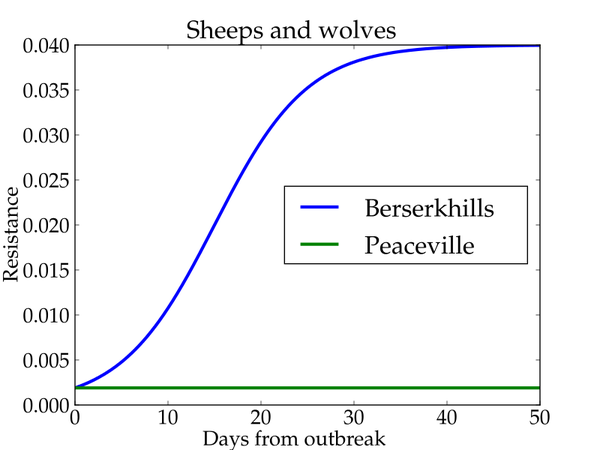Zombies

If I have to explain what differential equations are, I always use the zombie apocalypse as an example. People seem to understand that better than the popular Lotka-Volterra equations modeling predator-prey dynamics, despite the fact that the zombie equations of Munz et al. are much more complicated. Well, to be eaten alive by an undead is just much more scary than being eaten by, say, a lion.

Both the Lotka-Volterra and the Munz models constitute a system of nonlinear coupled differential equations for which no analytical solutions exist. We thus have to solve these equations numerically, which is possible, for example, by pylab.

The basic code to solve the Munz model is simple and short:

 1 2 3 4 5 6 7 8 9 10 11 12 13 14 15 16 17 18 19 20 21 22 23 24 25 26 27 28 29 30 31 32 33 34 35 36 37 38 39 40 41 42 43 44 45 46 47 48 49 50 51 #!/usr/bin/env python # -*- coding: utf-8 -*- # zombie apocalypse modeling from pylab import * from scipy.integrate import odeint params = {'text.usetex': True,'font.family': 'serif','font.size': 20} rcParams.update(params) P = 10 # birth rate d = 0.0001 # natural death rate B = 0.0095 # infection rate G = 0.0001 # resurrection rate A = 0.02 # killing rate # solve the system dy/dt = f(y, t) def f(y, t): Si = y Zi = y Ri = y # the model equations (see Munz et al. 2009) f0 = P - B*Si*Zi - d*Si f1 = B*Si*Zi + G*Ri - Si*Zi f2 = d*Si + Si*Zi - G*Ri return [f0, f1, f2] # initial conditions S0 = 500. # initial population Z0 = 0 # initial zombie population R0 = 0.01*S0 # initial death population y0 = [S0, Z0, R0] # initial condition vector t = linspace(0, 50., 10000) # time grid # solve the DEs soln = odeint(f, y0, t) S = soln[:, 0] Z = soln[:, 1] R = soln[:, 2] # plot results figure() plot(t, S, linewidth=3, label='Living') plot(t, Z, linewidth=3, label='Zombies') xlabel('Days from outbreak') ylabel('Population') title('Zombies in Peaceville: Apocalypse') legend(loc=0) show()

The situation in Peaceville is discouraging. People are doomed:Do we stand any chance at all against zombies? But of course we do! With the right spirit we fight them back and eliminate them from the face of the earth: 😉What makes the difference is the resistance the people in Peaceville and Berserkhill offer:What do we learn from that? Even apparently lost fights can be won by determination.